# Postprocessing

Post processing is a great feature which allows you to:

• add new math channels in analyse mode
• modify existing math channels
• change the scaling of online or offline channels.

So you could store only the raw data and then in analyse perform all needed mathematics. There are several ways to perform math analysis on already acquired data. Let’s start with a simple one. To add a simple math channel like filter you select the channel from channel selector, right click and select Add math and then choose appropriate math.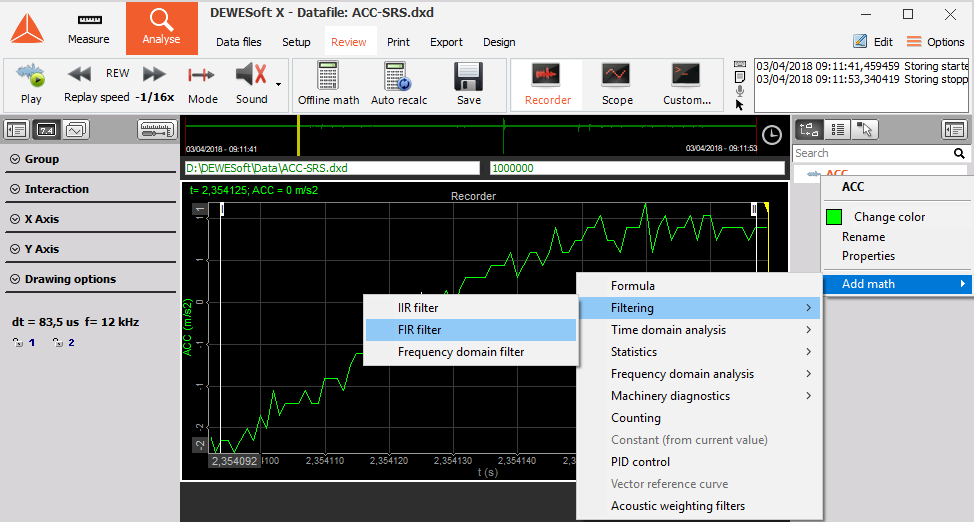In this example, an FIR filter was added. When a filter is set, data is automatically recalculated and put into the current display.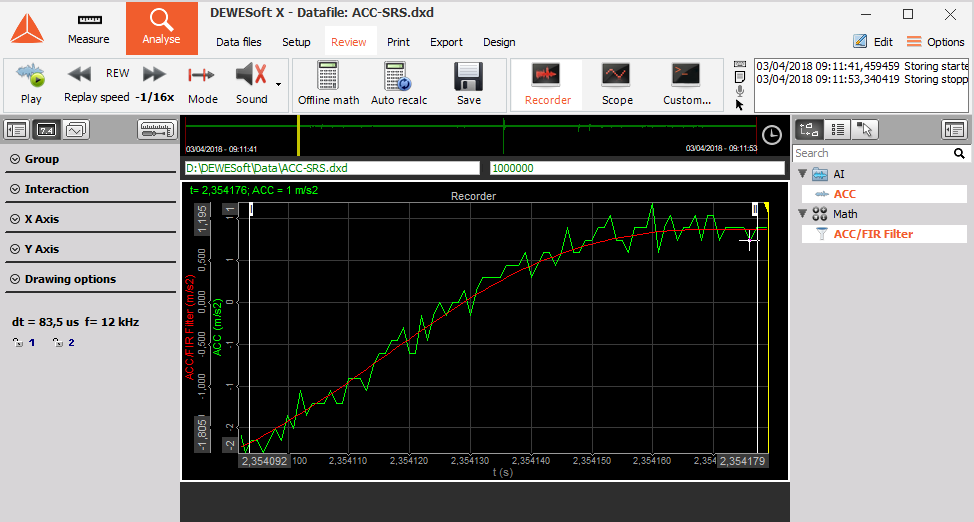Next, you can edit any math (either added during measurement or offline) and modify or delete it by clicking on the math channel and choosing Edit xxx math or Delete xxx math (in this case Edit FIR filter or Delete FIR filter).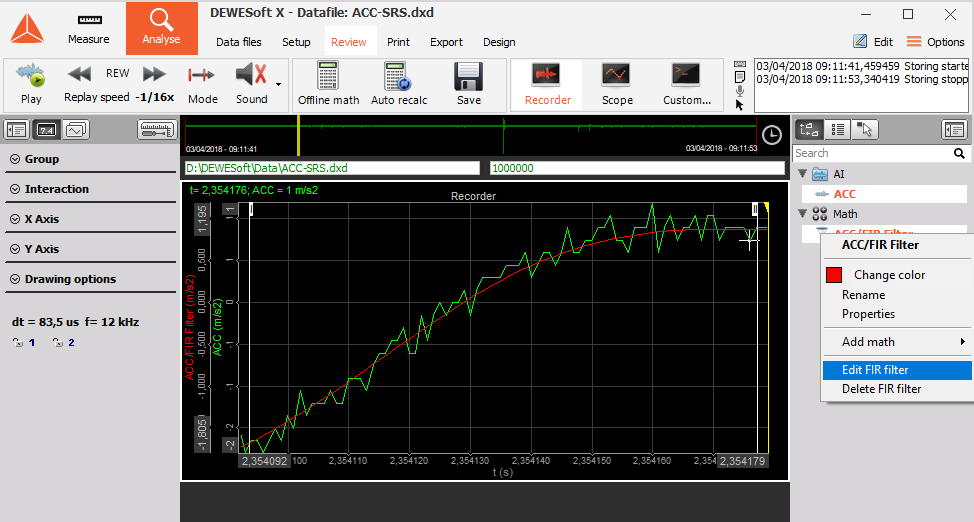A window of chosen math will pop up, allowing you to change calculation parameters. Upon closing the channels will be automatically recalculated.

NOTE: Data file will be recalculated only on the chosen time interval. To reduce the time needed to recalculate huge data files, you can zoom in and define all needed calculations on the small area and then zoom out, press Recalculate and get the cup of coffee when Dewesoft recalculates the whole file.

More complicated math can be defined in the math section by pressing Offline math button or Setup button from the top menu. Now you prepare math channels like in Measure -> Setup mode and add advanced analysis like Power, Order tracking, Order analysis and all others.

In our example let’s continue to add SRS (shock response spectrum) from the Setup menu.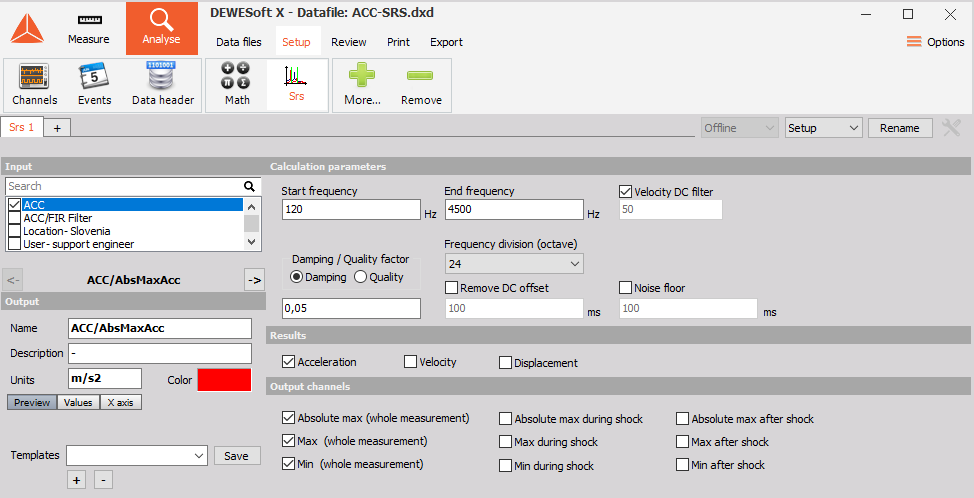When finished, go back to review. Please note that Offline math icon changed to Recalculate. SRS is typical offline math (since it requires double pass). Therefore select start and end for recalculation and press Recalculate.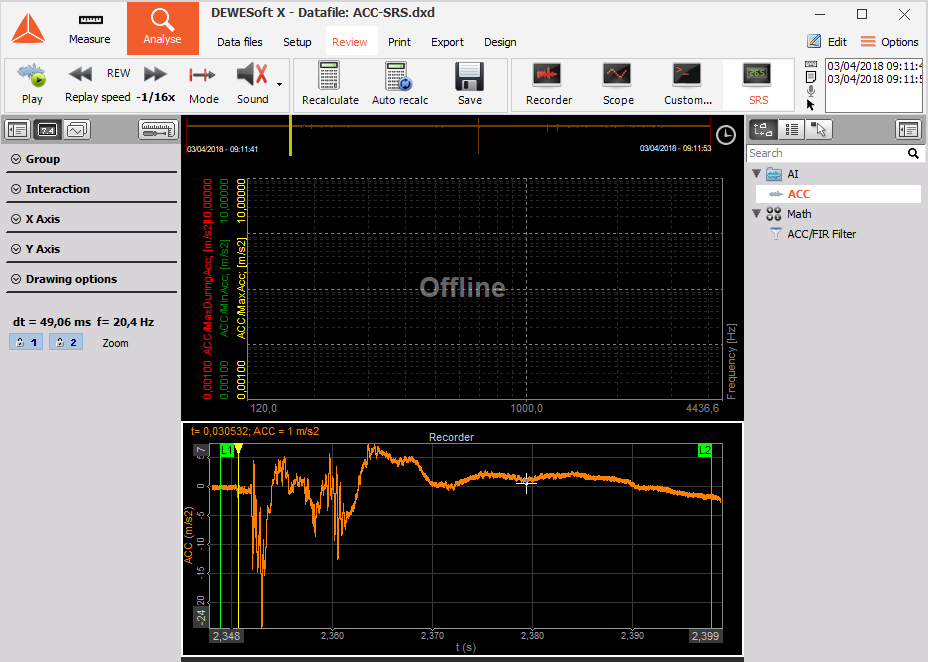SRS will be calculated. When recalculated, the button changes again to Offline math, notifying that we can go back to math and modify parameters to get perfect results. Please look also at the channel selector topic. You can change the colour, scale and offset of any channel.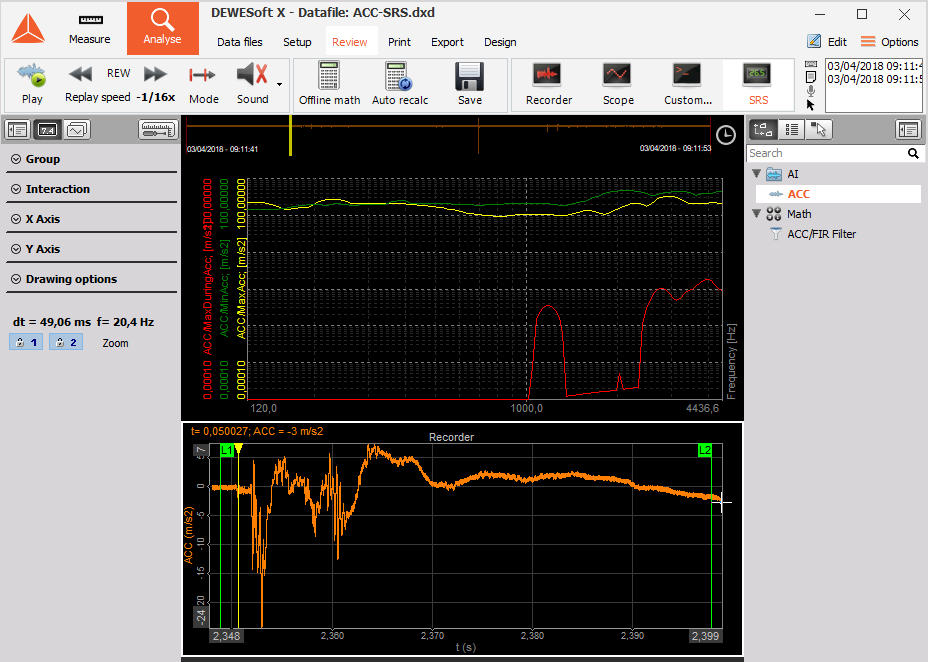NOTE: Lengthy calculations can be interrupted with Escape key on the keyboard.

You can also choose that Dewesoft automatically recalculates the data when zooming in or out. This is, for example, useful when searching for the absolute maximum for a specific time slice. You can save the results of post-processing by pressing Save button. If the data is not calculated or not calculated for the full time of the file, only the setup of all math and display channels will be stored. If the offline math is recalculated for the entire time of the file, also the recalculated results will be stored in the data file.